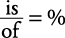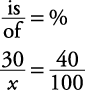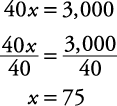## Percent

Here is an example of calculating percents in word problems.

##### Example 1

Thirty students are awarded doctoral degrees at the graduate school, and this number comprises 40% of the total graduate student body. How many graduate students were enrolled?

First, circle what you must find in the problem— how many graduate students. Now, in order to plug into the percentage equationtry rephrasing the question into a simple sentence. For example, in this case,

30 is 40% of what total?

Notice that the 30 sits next to the word is; therefore, 30 is the “is” number. 40 is the percent. Notice that what total sits next to the word of. Therefore, plugging into the equation,Cross multiplying,Therefore, the total graduate enrollment was 75 students.